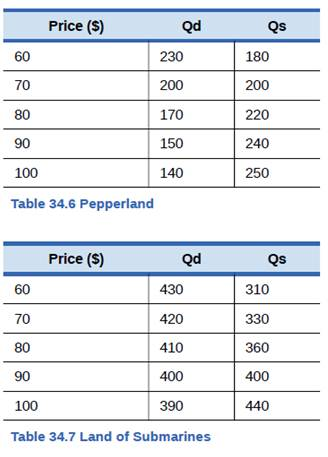Chapter 34, Problem 57P### Principles of Economics 2e

2nd Edition
Steven A. Greenlaw; David Shapiro
ISBN: 9781947172364

#### Solutions

Chapter
Section### Principles of Economics 2e

2nd Edition
Steven A. Greenlaw; David Shapiro
ISBN: 9781947172364
Textbook Problem

# The country of Pepperland exports steel to the Land of Submarines. Information for the quantity demanded (Qd) and quantity supplied (Qs) in each country, in a world without trade, are given in Table 34.6 and Table 34.7 .a. What would be the equilibrium price and quantity in each country in a world without trade? How can you tell?b. What would be the equilibrium price and quantity in each country if trade is allowed to occur? How can you tell?C. Sketch two supply and demand diagrams, one for each country, in the situation before trade.d. On those diagrams, show the equilibrium price and the levels of exports and imports in the world after trade.e. If the Land of Submarines imposes an anti- dumping import quota of 30, explain in general terms whether it will benefit or injure consumers and producers in each country.f. Does your general answer change if the Land of Submarines imposes an import quota of 70?

a)

To determine

The equilibrium price and quantity in Pepperland and Land of Submarines without trade is to be determined.

Explanation

The pre-trade equilibrium is defined as the situation where the market forces of demand and supply are balanced. That is, without trade equilibrium would be where the quantity demanded of steel and quantity supplied of steel are equal...

b)

To determine

The equilibrium price and quantity in each country after trade is to be determined.

c)

To determine

The pre-trade supply and demand graphs for each country is to be determined

d)

To determine

The price, export and import levels after trade are to be determined.

e)

To determine

The effect of an anti-dumping import quota on the producers and consumers of each country is to be determined.

f)

To determine

Whether the effect of an anti-dumping import quota of 70 units is different from an import quota of 30 units is to be determined.

### Still sussing out bartleby?

Check out a sample textbook solution.

See a sample solution

#### The Solution to Your Study Problems

Bartleby provides explanations to thousands of textbook problems written by our experts, many with advanced degrees!

Get Started

#### Find more solutions based on key concepts